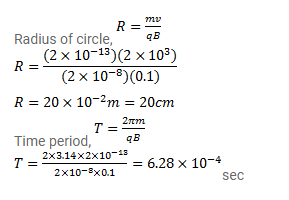# A particle having a charge

Question:

A particle having a charge $2.0 \times 10^{8} \mathrm{C}$ and a mass of $2.0 \times 10^{-10} \mathrm{~g}$ is projected with a speed of $2.0 \times 10^{3} \mathrm{~m} / \mathrm{s}$ in a region having a uniform magnetic field of $0.10 \mathrm{~T}$. The velocity is perpendicular to the field. Find the radius of the circle formed by the particle and also the time period.

Solution: